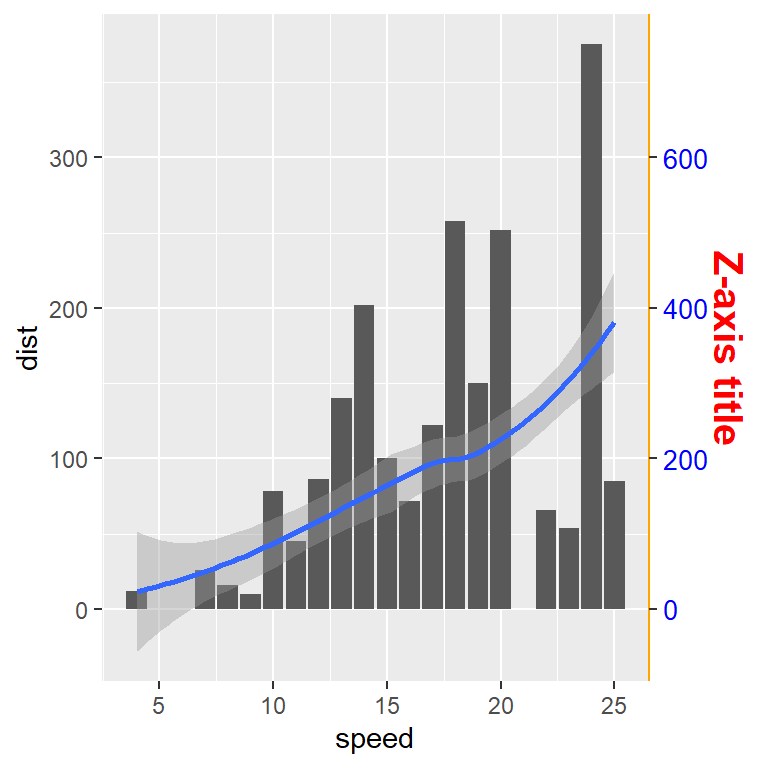# ggplot2 axis titles, labels, ticks, limits and scales

## Sample plots

In this tutorial we are going to use the following sample plots and then customize their axes. Note that the axes can be continuous if the variable is numerical, discrete for categories or groups or date if the axis represents dates.

The following sample plot is a box plot by group has a discrete X-axis and a continuous Y-axis:

``````# install.packages("ggplot2")
library(ggplot2)

# Sample data
x <- droplevels(chickwts\$feed[1:36])
levels(x) <- LETTERS[1:3]
y <- chickwts\$weight[1:36]
df <- data.frame(x = x, y = y)

# Plot
p <- ggplot(df, aes(x = x, y = y)) +
stat_boxplot(geom = "errorbar",
width = 0.25) +
geom_boxplot(fill = "dodgerblue1",
colour = "black",
alpha = 0.5,
outlier.colour = "tomato2")
p``````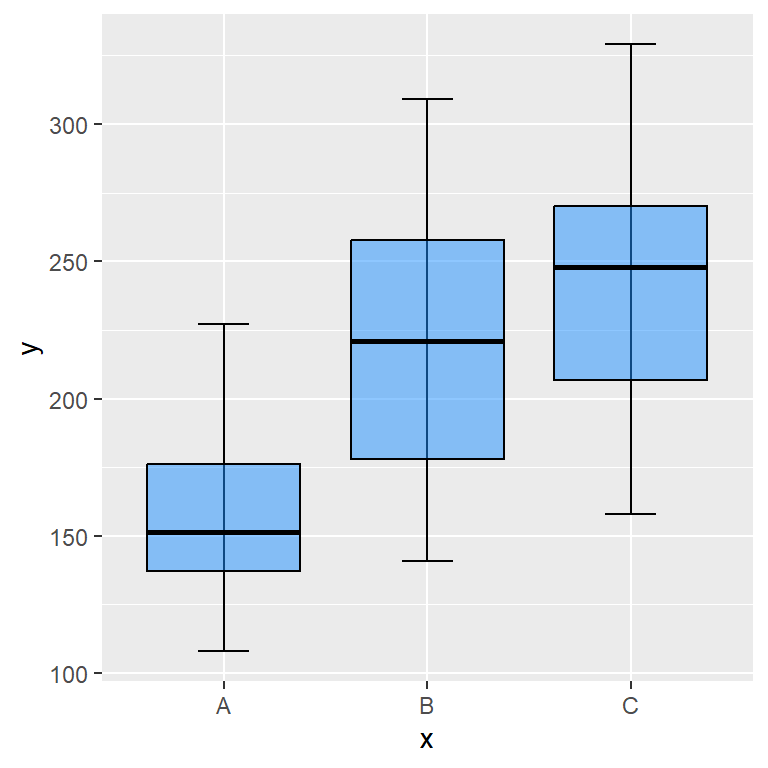The other sample plot we are going to use is a scatter plot, so both axis are continuous.

``````# install.packages("ggplot2")
library(ggplot2)

# Sample plot with continuous axes
ggplot(cars, aes(x = speed, y = dist)) +
geom_point() ``````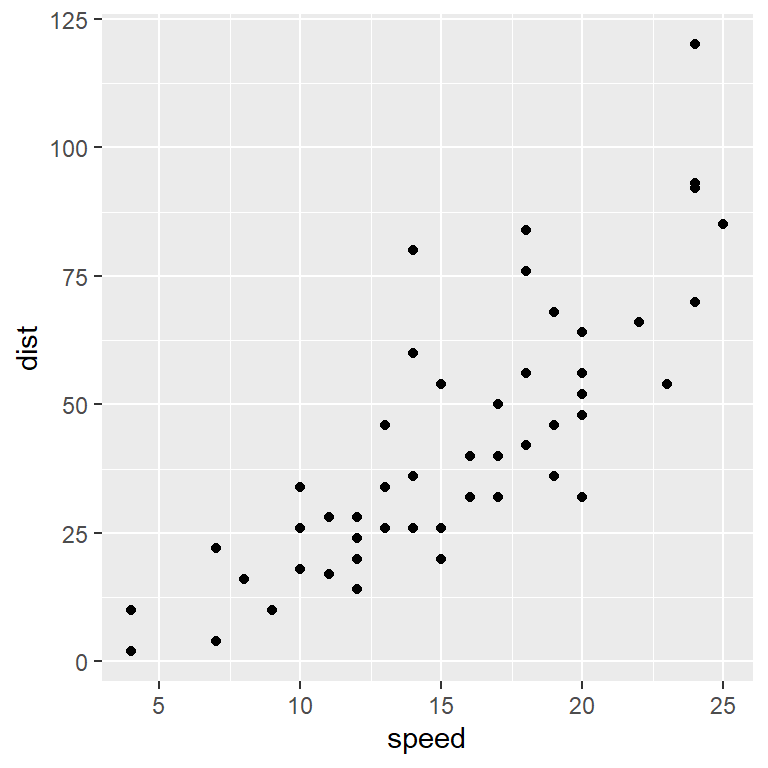The sample plot with the box plots has a discrete X-axis and a continuous Y-axis, so you might need to adapt some functions of the examples of this tutorial depending on your data. For instance, if the X-axis of your plot is continuous and you want to change the labels you will need to use `scale_x_continuous` instead of `scale_x_discrete`.

## Axis titles

### `labs` function

By default, the axis titles are the name of the variables assigned to each axis inside `aes`, but you can change the default axis labels with the `labs` function as follows.

``p + labs(x = "X-axis title", y = "Y-axis title")``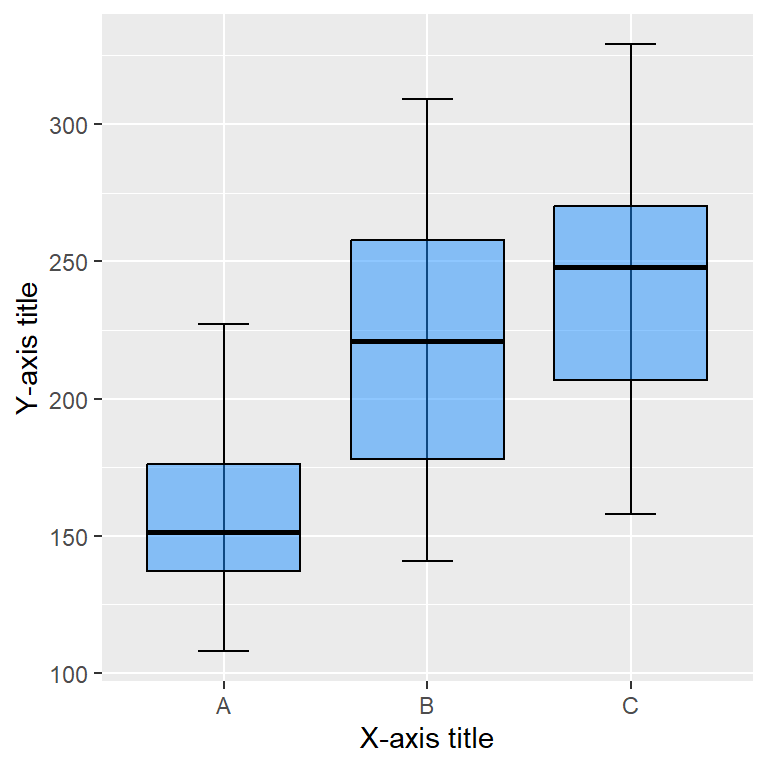### `xlab` and `ylab` functions

Alternatively, you can use `xlab` and `ylab` functions to set the axis titles individually.

``````p + xlab("X-axis title") +
ylab("Y-axis title")``````### Size, style and colors

The style of the axis titles can be modified through the `axis.title` component of the `theme` function. You will need to pass an `element_text` and customize the style with the corresponding arguments, such as `size`, `color` or `face`.

``````p + labs(x = "X-axis title", y = "Y-axis title") +
theme(axis.title = element_text(size = 15,
color = "blue",
face = "bold"))``````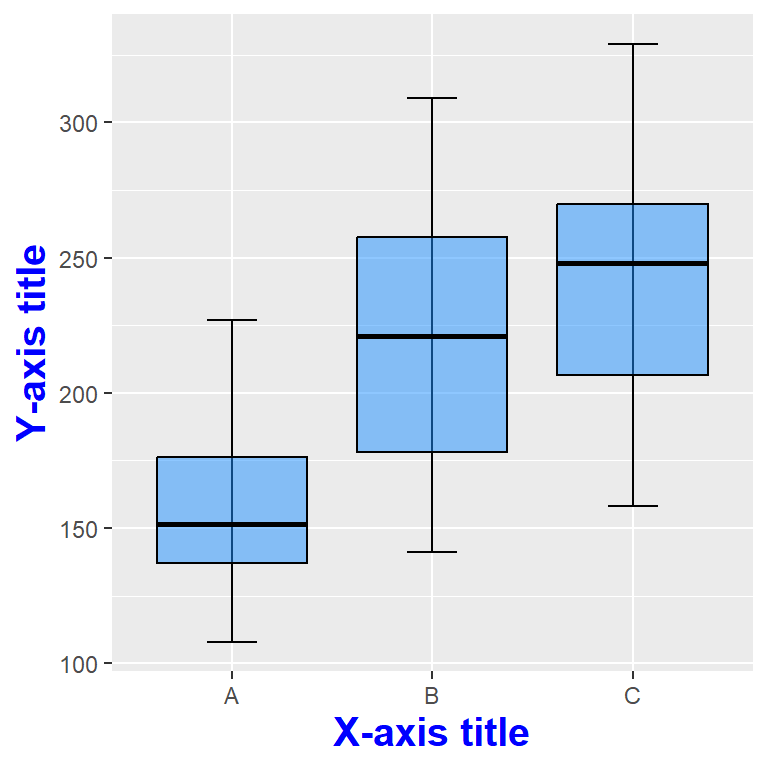Note that you can also customize each axis title appending `.x` or `.y` to `axis.title` as follows. Recall that if you don’t specify one of them it will inherit the default aesthetics.

``````p + labs(x = "X-axis title", y = "Y-axis title") +
theme(axis.title.x = element_text(size = 15,
color = "blue",
face = "bold"),
axis.title.y = element_text(size = 10,
color = "red",
face = "italic"))``````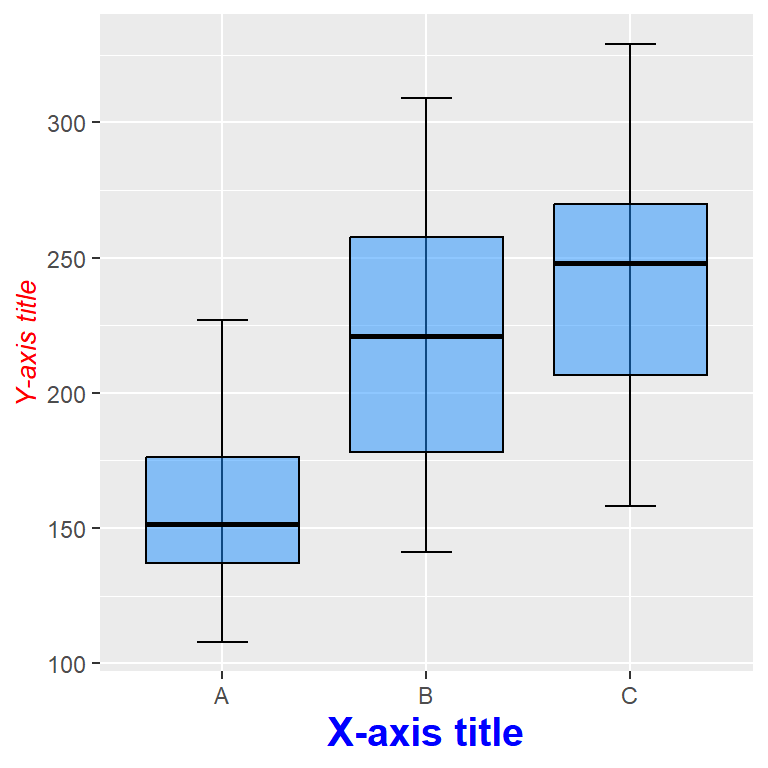### Remove axis titles

Remove both axis titles

Setting a theme component to `element_blank()` will remove the corresponding element. In order to remove the axis titles you can pass the `element_blank` function to the `axis.title` component, even if you have already specified the titles, as long as you don’t add them again.

``````p + xlab("X-axis title") +
ylab("Y-axis title") +
theme(axis.title = element_blank())``````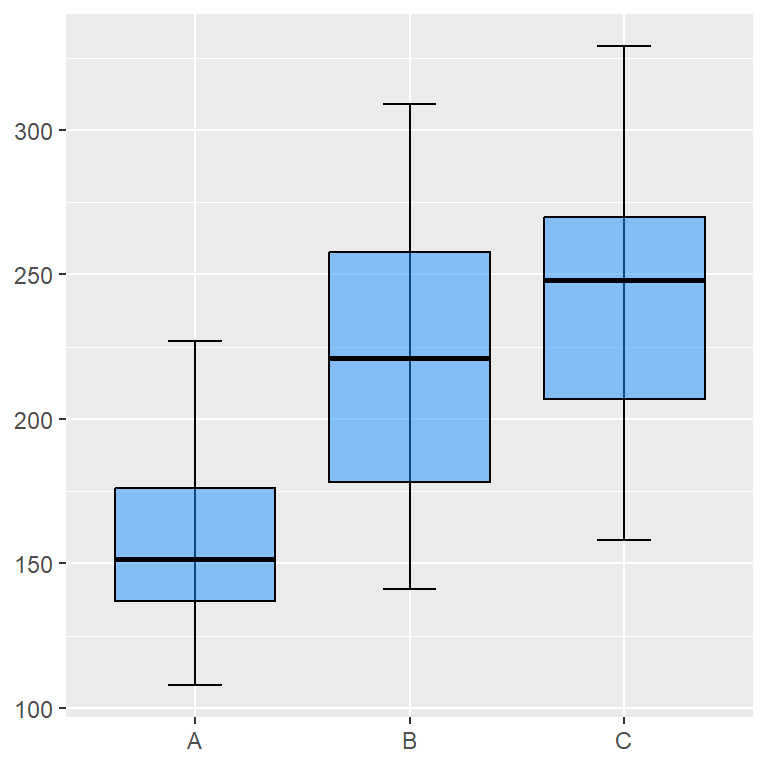Remove one of the axis titles

If you append `.x` or `.y` to the `axis.title` component you can remove only one of the axis titles. This is, you can remove the X-axis title setting `axis.title.x = element_blank()` and the Y axis title with `axis.title.y = element_blank()`.

``````p + xlab("X-axis title") +
ylab("Y-axis title") +
theme(axis.title.x = element_blank())``````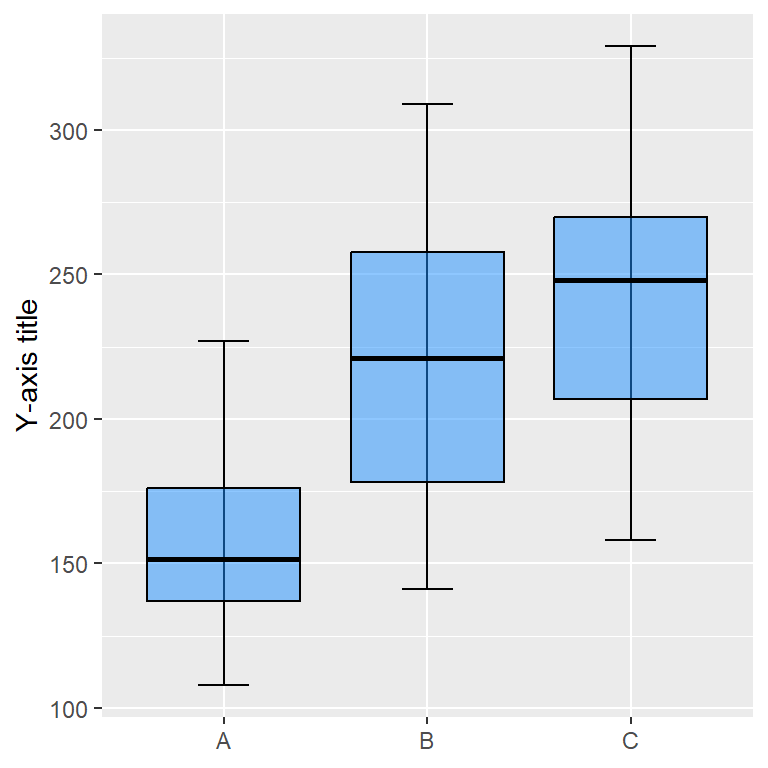## Axis labels

Each axis will have automatic axis labels or texts. For instance, the default axis labels for the Y-axis of our example ranges from 100 to 300 with a step size of 50 and the labels of the X-axis are the names of the different groups (A, B and C). These labels can be customized with `scale_(x|y)_continuous` if the axis (x or y) is continuous, `scale_(x|y)_discrete` if the axis is discrete or other variants, such as `scale_(x|y)_datetime`, `scale_(x|y)_date`, `scale_(x|y)_reverse`, `scale_(x|y)_log10` etc.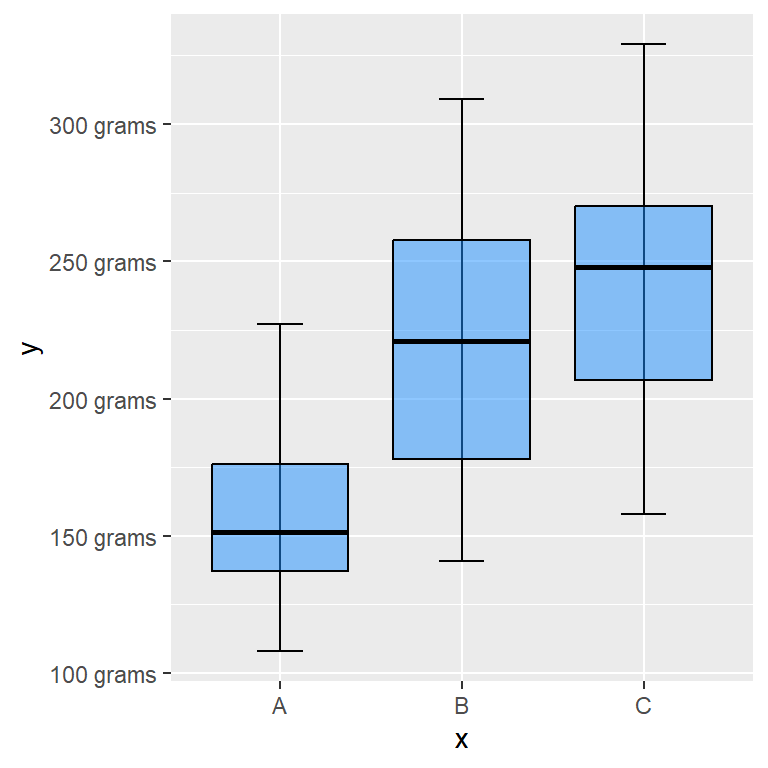Custom Y-axis labels

As the Y-axis of our sample plot is continuous we can use the `scale_y_continuous` function to customize it. The `labels` argument is the one used to customize the labels, where you can input a vector with the new labels or a custom labeller function as in the example below.

``````# Custom Y-axis labels
labels <- function(x) {
paste(x, "grams")
}

p + scale_y_continuous(label = labels)``````

The length of the vector passed to `labels` must equal the number of breaks or ticks of the axis. See the following section for further clarification.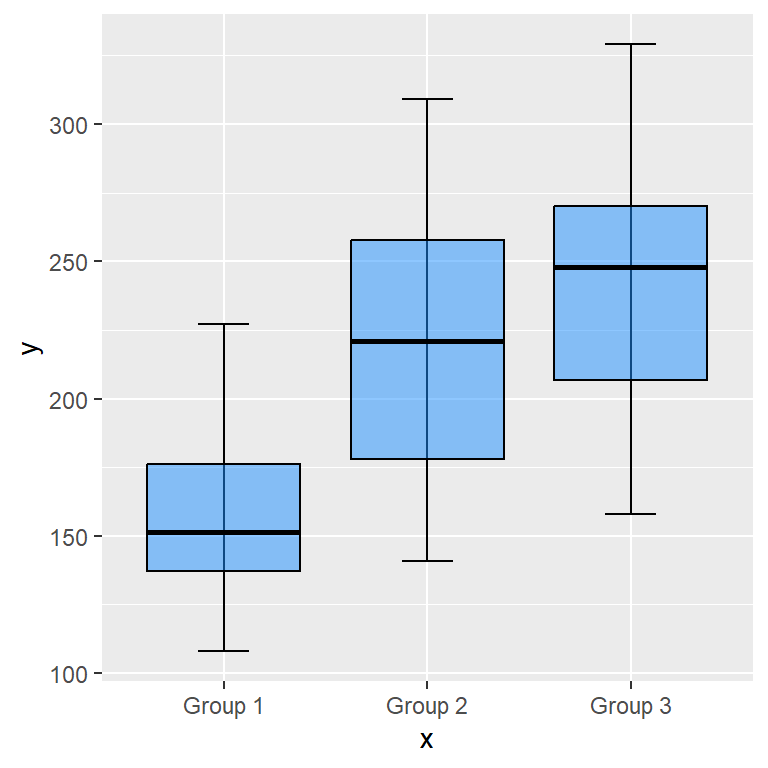Custom X-axis labels

As the X-axis of our sample plot represents groups, is discrete, so we can use the `scale_x_discrete` function to customize the labels for the X-axis. You can pass a function or a vector with the new names to `labels`.

``````# Custom X-axis labels
labels <- c("Group 1", "Group 2", "Group 3")

p + scale_x_discrete(label = labels)``````

### Size, colors and style of the axis labels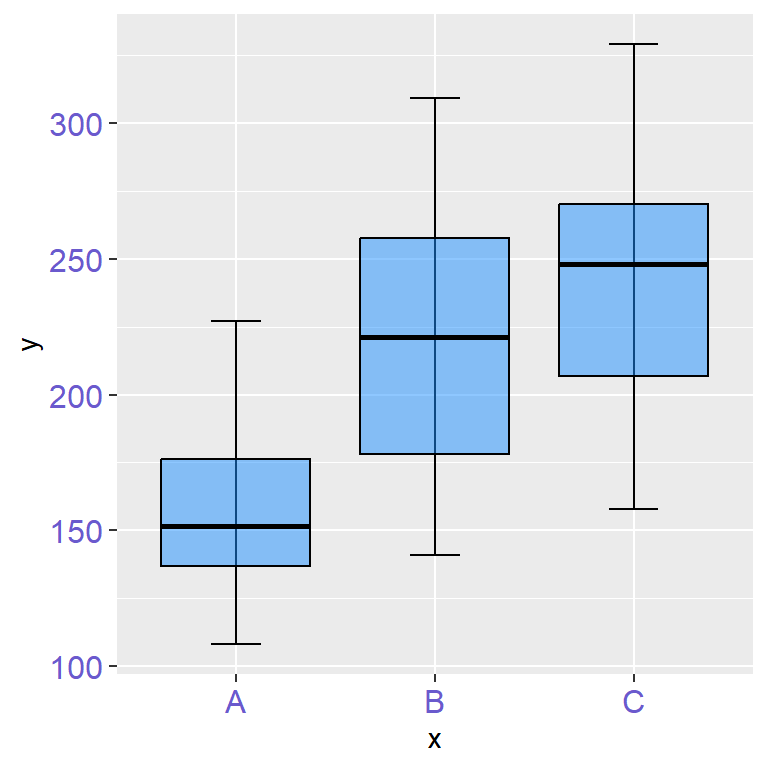Color of the axis texts

With the `axis.text` component of the `theme` function you can control the style of the axis labels. If you pass an `element_text` you will be able to customize the style of the texts with its arguments, such as its color, size, angle…

``````p +
theme(axis.text = element_text(color = "slateblue",
size = 12))``````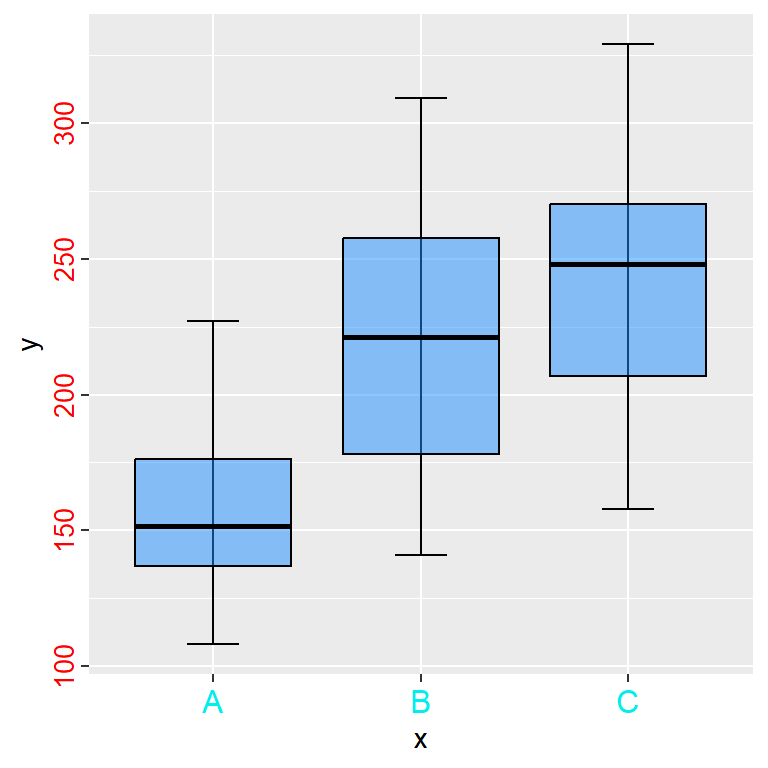Different color for each axis text

Recall that you can apply a different styling for each axis just appending `.x` or `.y` to the corresponding component, this is, with `axis.text.x` and with `axis.text.y`.

``````p +
theme(axis.text.x = element_text(color = "cyan2",
size = 12),
axis.text.y = element_text(color = "red",
size = 10,
hjust = 1))``````

## Axis ticks (breaks)

The ticks are the marks that divide the axes. These marks are adjusted automatically by ggplot2 based on your data, but you can also customize them. It is possible to increase or decrease the number of ticks, customize its style, increase its size or remove them.

Increase the number of ticks

The number of ticks can be customized passing a vector with the desired values to the `breaks` argument of the `scale_y_continuous` function. In the following example we are passing a sequence of values that ranges from 120 to 320 with step size of 20.

``````p +
scale_y_continuous(breaks = seq(120, 320, by = 20))``````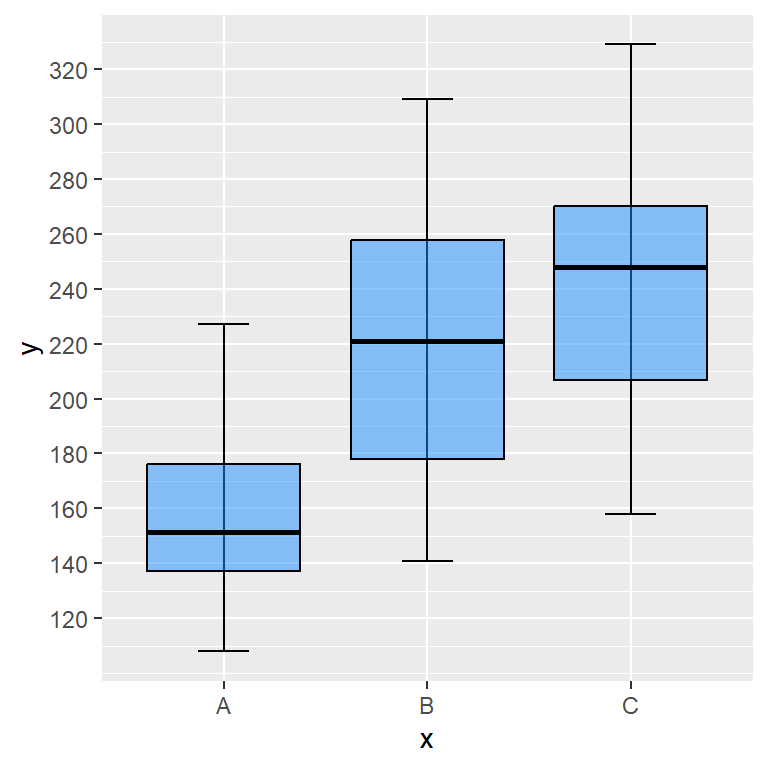Increase the size of the ticks

The `theme` function provides a component named `axis.ticks.length` to increase or decrease the size of the axis ticks. In order to accomplish that you will need to pass the `unit` function and specify the desired size in the unit you want. Recall to use `axis.ticks.length.x` or `axis.ticks.length.y` to customize only one axis ticks.

``````p +
theme(axis.ticks.length  = unit(0.5, "cm"))``````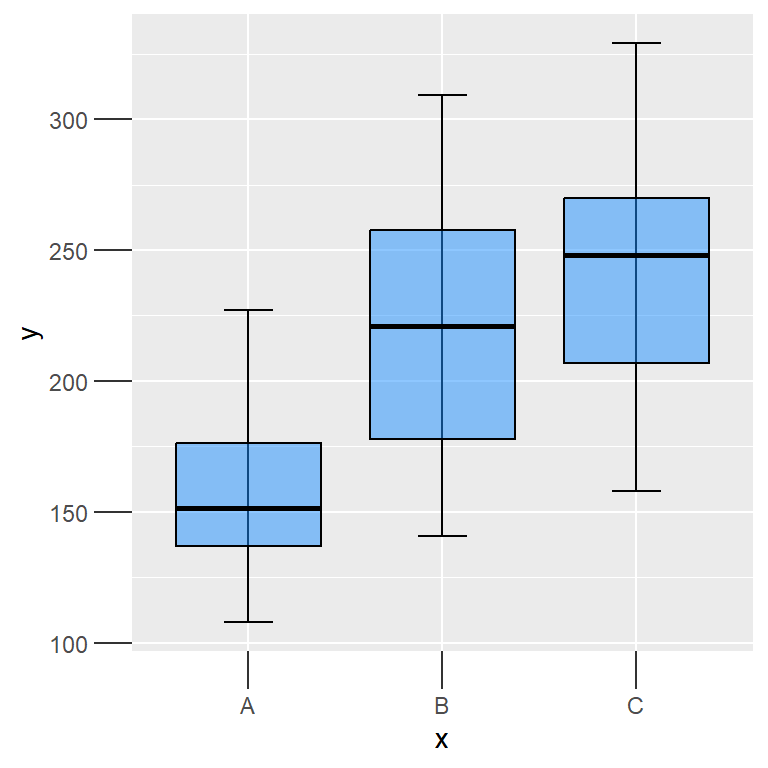Modify the style of the ticks

You can customize the style of the ticks passing an `element_line` to the `axis.ticks` component of the `theme` function, specifying the desired color, size, line style, etc. Recall to append `.x` or `.y` to customize only one axis.

``````p +
theme(axis.ticks = element_line(color = 2,
linewidth = 2))``````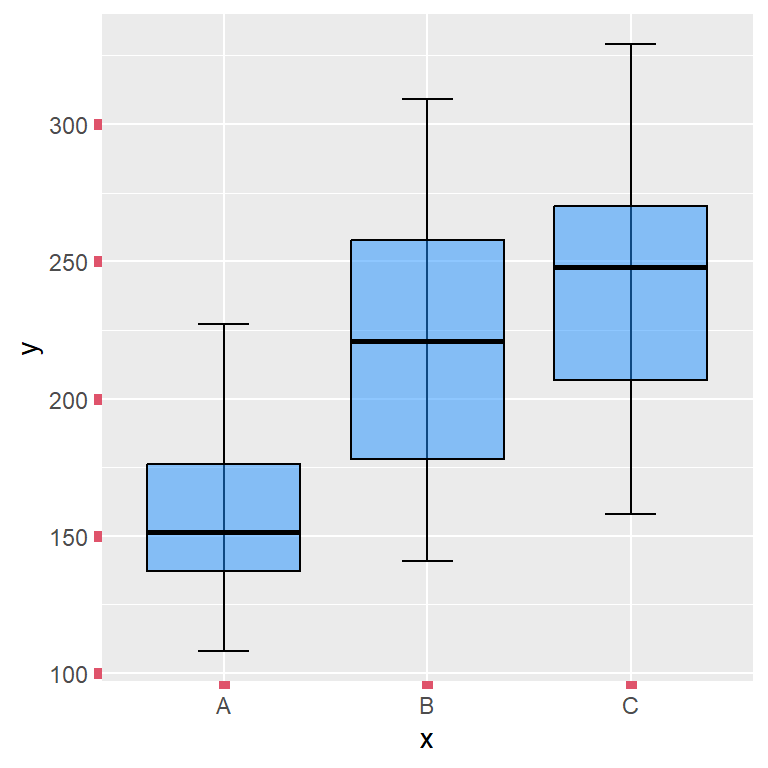Remove the ticks

As with other components, passing the `element_blank` function to the `axis.ticks` component will remove the ticks from both axis. If you want to remove the ticks only for one axis pass the function to `axis.ticks.x` or to `axis.ticks.y`.

``````p +
theme(axis.ticks = element_blank())``````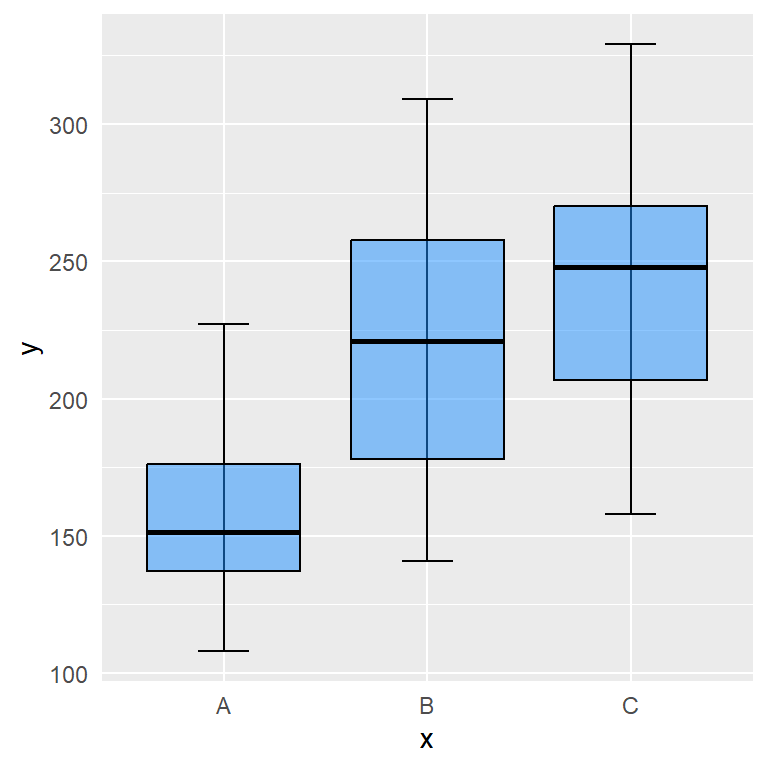The `theme` function also provides the `axis.ticks.x.top`, `axis.ticks.x.bottom`, `axis.ticks.y.left`, `axis.ticks.y.right`, `axis.ticks.length.x`, `axis.ticks.length.x.top`, `axis.ticks.length.x.bottom`, `axis.ticks.length.y`, `axis.ticks.length.y.left` and `axis.ticks.length.x.right` components.

## Axis limits

The range or limits of the axes are adjusted automatically by ggplot2 based on the range of your data. Nonetheless, you can override the automatic settings and customize the limits to zoom in or zoom out or to subset the data.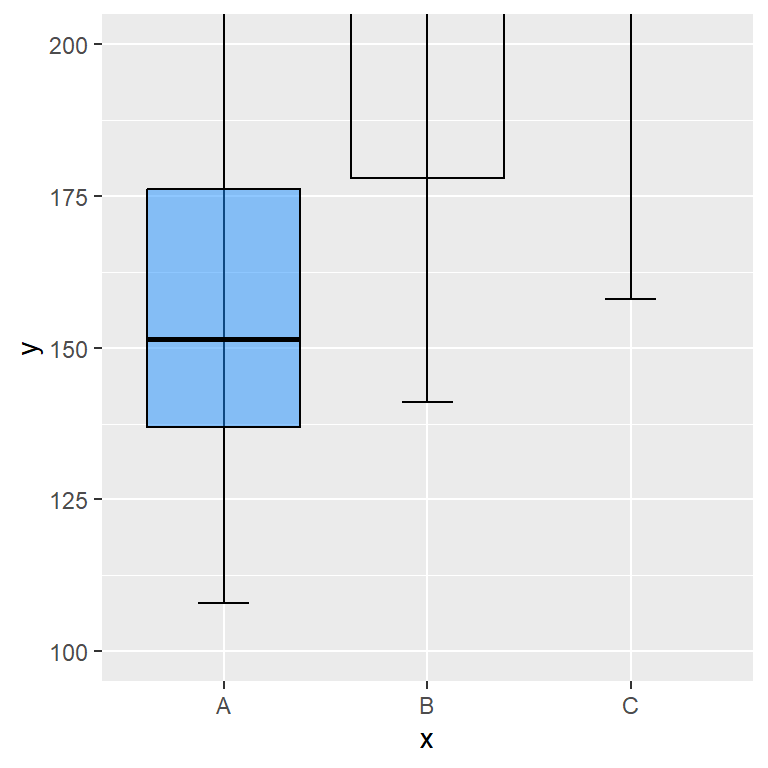coord_cartesian (zooming in)

In case you want to zoom in or zoom out the plot without removing data you will need to use the `ylim` and `xlim` arguments of the `coord_cartesian` function.

``````p +
coord_cartesian(ylim = c(100, 200))``````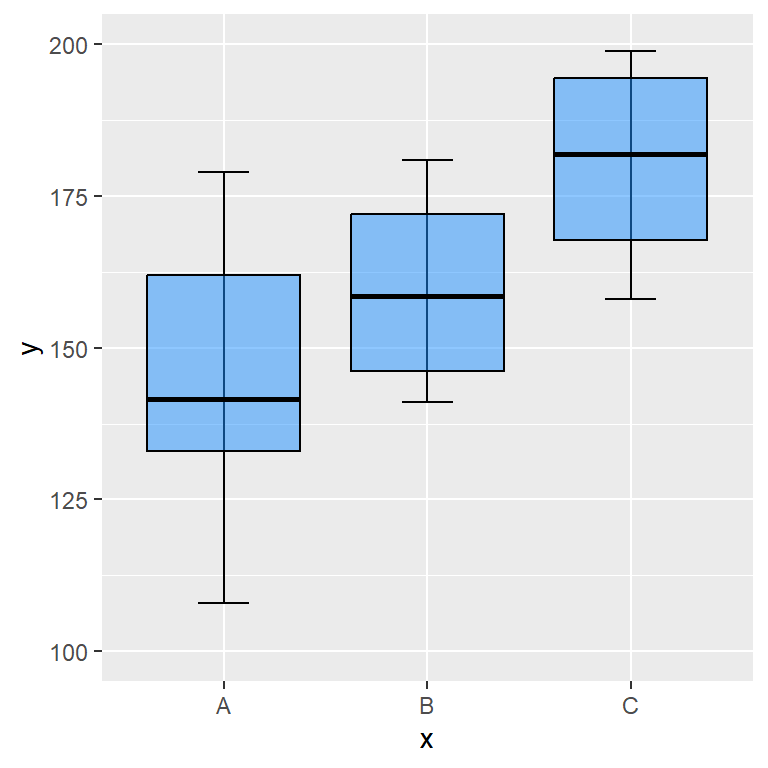ylim and xlim functions (subsetting)

The `xlim` and `ylim` functions subset the data to the specified range and then calculate the stats again. It is really important to understand that these functions doesn’t zoom in, they subset the data.

``````p +
ylim(c(100, 200))``````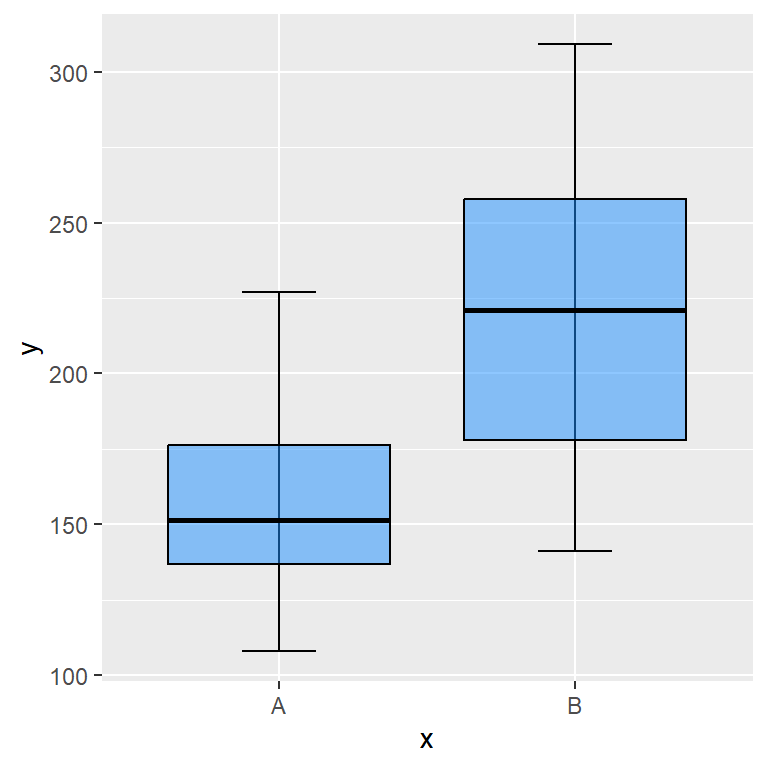Note that if one of your axes is discrete you can subset the data passing a vector with the names of the desired groups.

``````p +
xlim(c("A", "B"))``````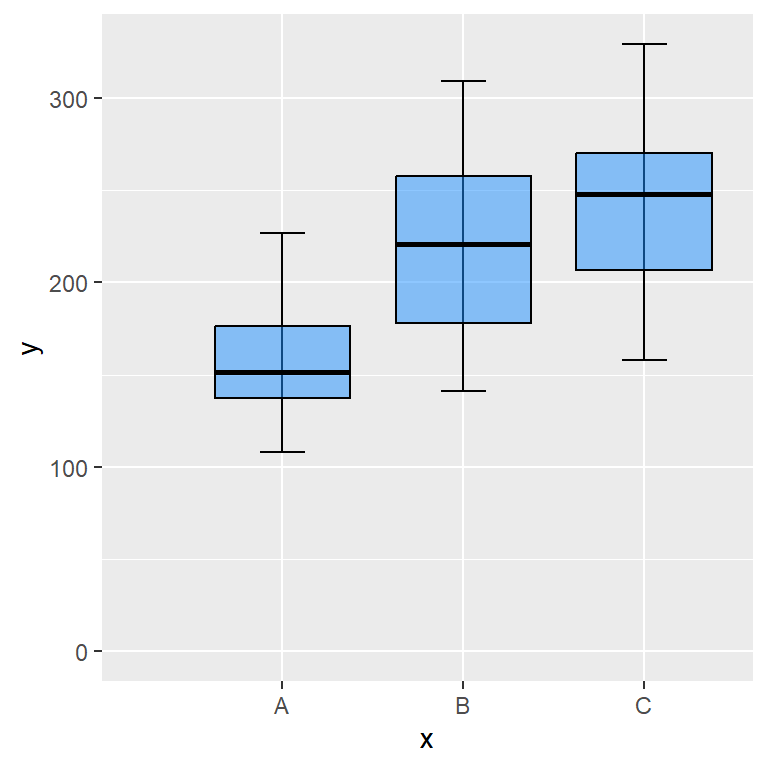Start at the origin

You can force the plot to start at the origin with the `expand_limits` function, which will force the axis to include the value or values passed to the `x` or `y` arguments of the function.

``````p +
expand_limits(x = 0, y = 0)``````

## Axis scales

The scales of the axes are automatically set by ggplot2 based on the data, but sometimes you might need to customize them. For instance, if the numbers are too big, ggplot2 will use a scientific notation format for the axis labels and you might want to remove it, or you might want to customize the decimal or thousands separator, change the format of the axis and treat them as currencies or maybe you want to transform the scale into a logarithmic scale.

Remove the scientific notation

If the numbers of the axis are too big, ggplot2 will show them by default in scientific notation. However, you can use the function from the following block of code and set the new labels without scientific notation and with marks.

``````# Function to set numbers with marks and without scientific notation
marks_no_sci <- function(x) format(x, big.mark = ".", decimal.mark = ",", scientific = FALSE)

ggplot(cars, aes(x = speed, y = dist * 1000000)) +
geom_point() +
scale_y_continuous(labels = marks_no_sci)``````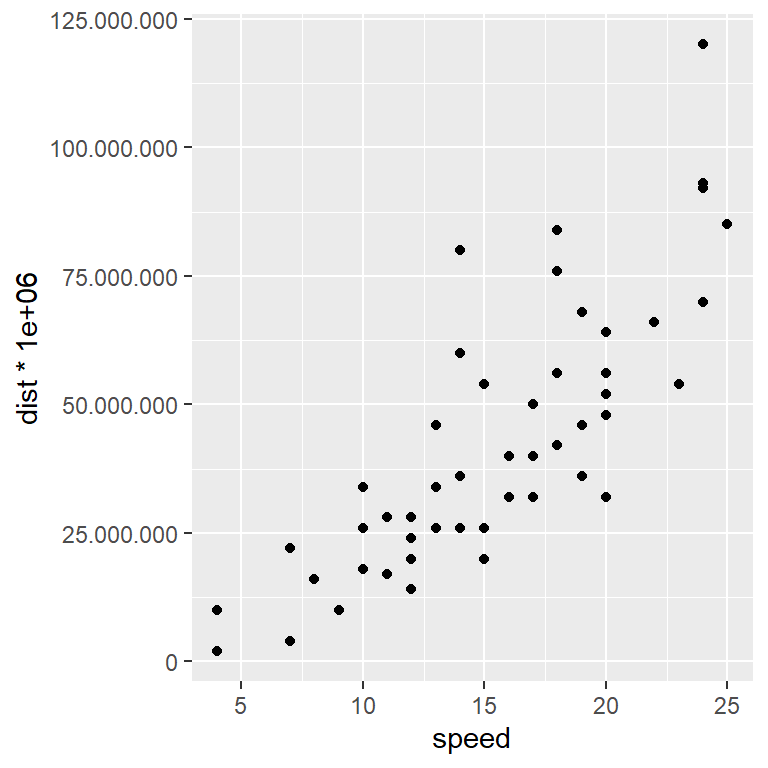Remove the scientific notation with `scales`

The `scales` package is a package that provides automatic methods to set breaks and labels for the axes. In order to remove the scientific notation, you just need to pass the function you want to use (`label_number` or `label_comma` in this scenario) to `labels`.

``````# install.packages("scales")
library(scales)

ggplot(cars, aes(x = speed, y = dist * 1000000)) +
geom_point() +
scale_y_continuous(labels = label_number())``````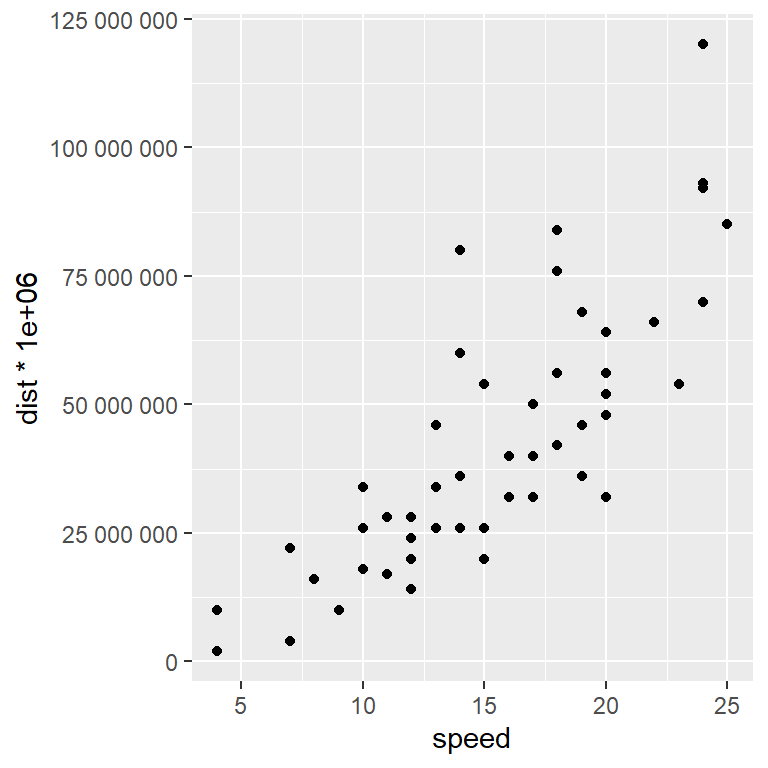Other alternative is to set something like `options(scipen = -10)`. Recall that the integer passed is based on a penalty mechanism to decide whether to print in scientific notation or not.

Dollar format

If your axis represents dollars you can use the `label_dollar` function to treat the axis as currency and hence a dollar sign will appear as prefix of the number.

``````# install.packages("scales")
library(scales)

ggplot(cars, aes(x = speed, y = dist)) +
geom_point() +
scale_y_continuous(labels = label_dollar())``````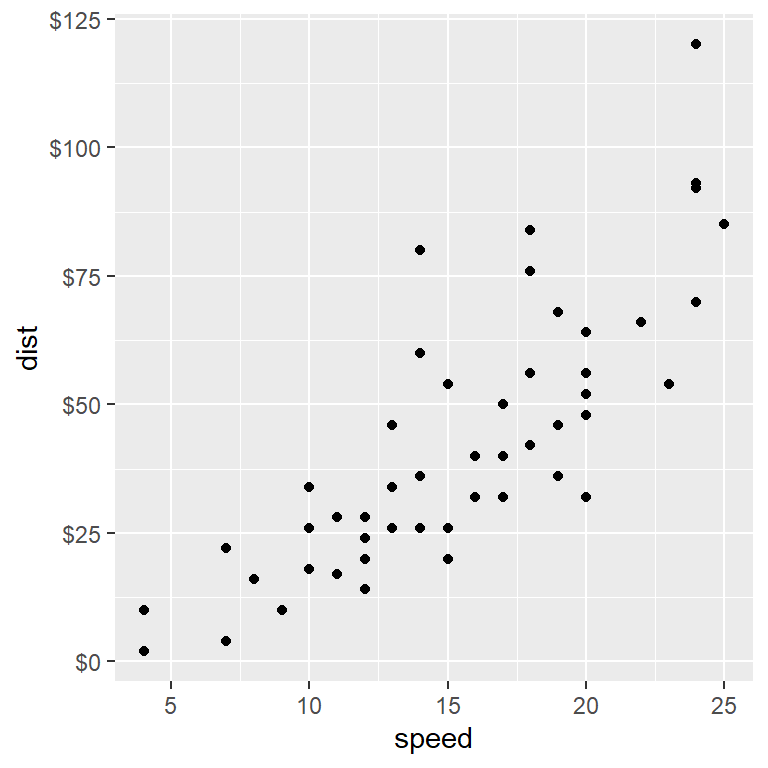Axis with other currencies (euros, pesos, yens …)

If you need other currency, such as euros, pesos or yens, you can also use the `label_dollar` function, but you will need to specify the desired symbol inside the `suffix` and `prefix` arguments. Note that by default, `prefix = "\$"`.

``````# install.packages("scales")
library(scales)

ggplot(cars, aes(x = speed, y = dist)) +
geom_point() +
scale_y_continuous(labels = label_dollar(suffix = "€", prefix = ""))``````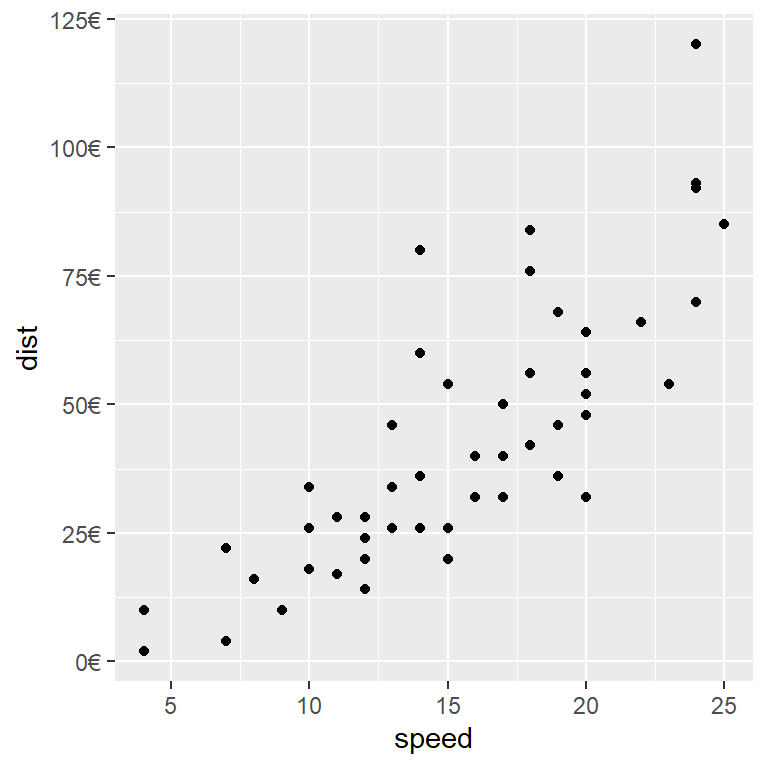Other interesting scales provided by `scales` are `label_bytes`, `label_date`, `label_date_short`, `label_math`, `label_percent`, `label_pvalue` or `label_scientific`, among others.

Scale log 10

ggplot2 also provides some functions for scale transformations, such as `scale_y_log10` or `scale_x_log10` that will transform the axis into a logarithmic scale.

``````ggplot(cars, aes(x = speed, y = dist)) +
geom_point() +
scale_y_log10()``````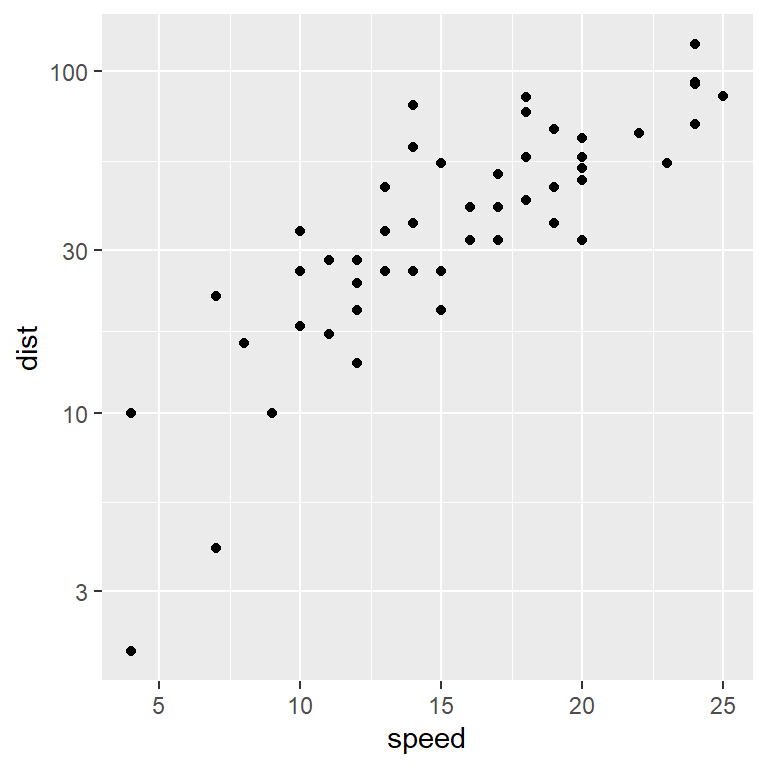Square root scale

The `scale_y_sqrt` and `scale_x_sqrt` functions will transform the corresponding axis applying the square root to its values.

``````ggplot(cars, aes(x = speed, y = dist)) +
geom_point() +
scale_y_sqrt()``````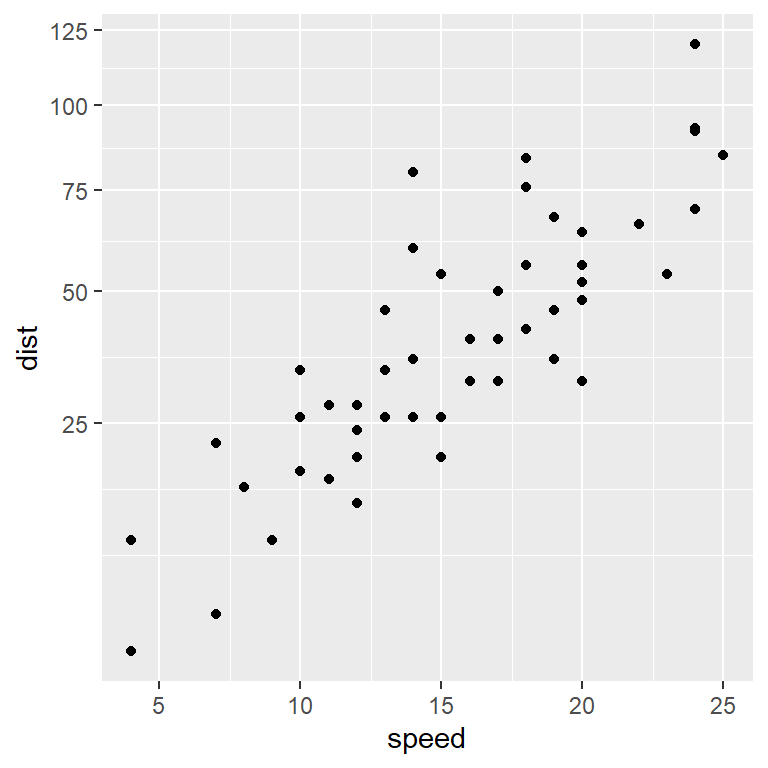Reversed scale

The `scale_y_reverse` and `scale_x_reverse` functions will reverse the Y and X axis, respectively.

``````ggplot(cars, aes(x = speed, y = dist)) +
geom_point() +
scale_y_reverse()``````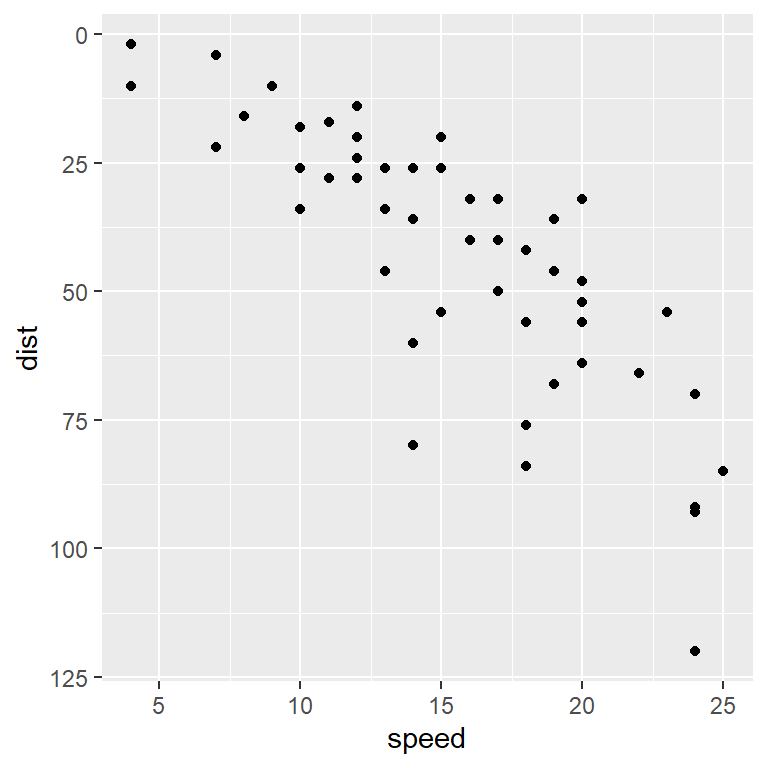## Style of the axis lines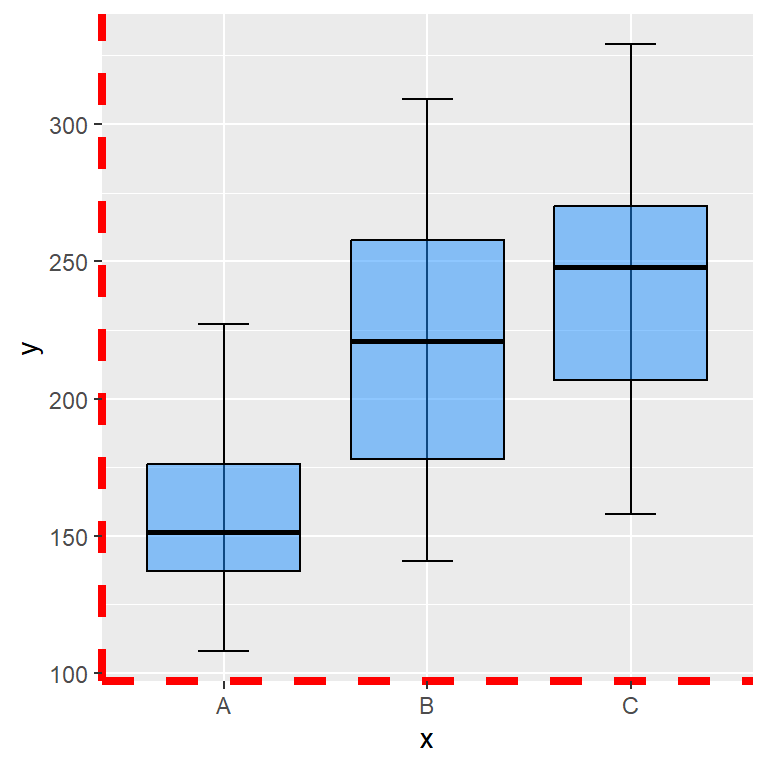The default ggplot2 theme doesn’t show axis lines, but if you are using other theme or you want to add lines to the axis you can pass an `element_line` to the `axis.line` component of the `theme` function. Then, you can set the width, color, line type, etc, or leave the default arguments.

``````p +
theme(axis.line = element_line(color = "red",
linewidth = 1.5,
linetype = 2))``````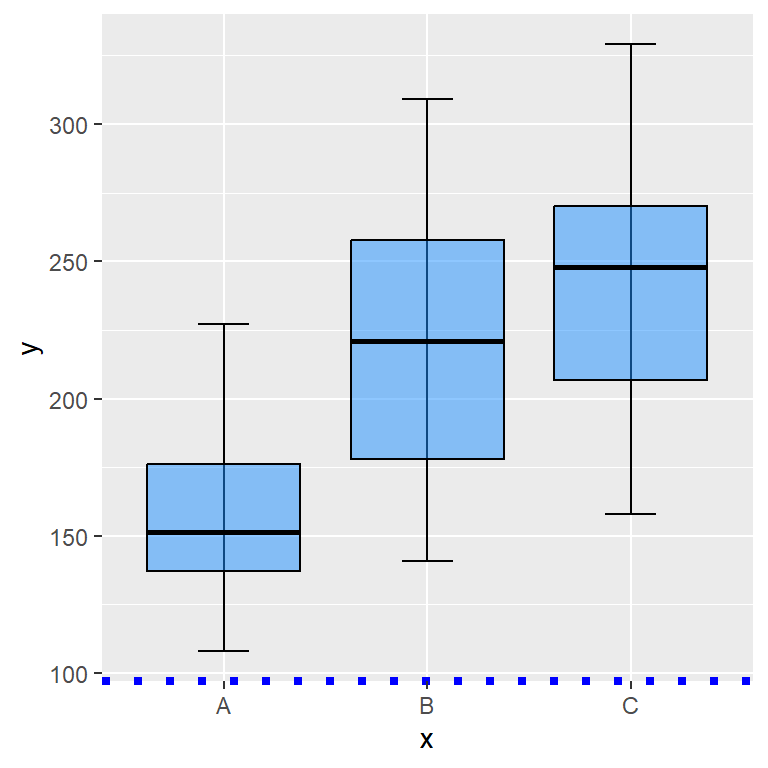Recall that if you want to customize or set only one axis you will need to append `.x` or `.y` to the `axis.line` component to customize the X-axis or Y-axis line, respectively.

``````p +
theme(axis.line.x = element_line(color = "blue",
linewidth = 1.5,
linetype = 3))``````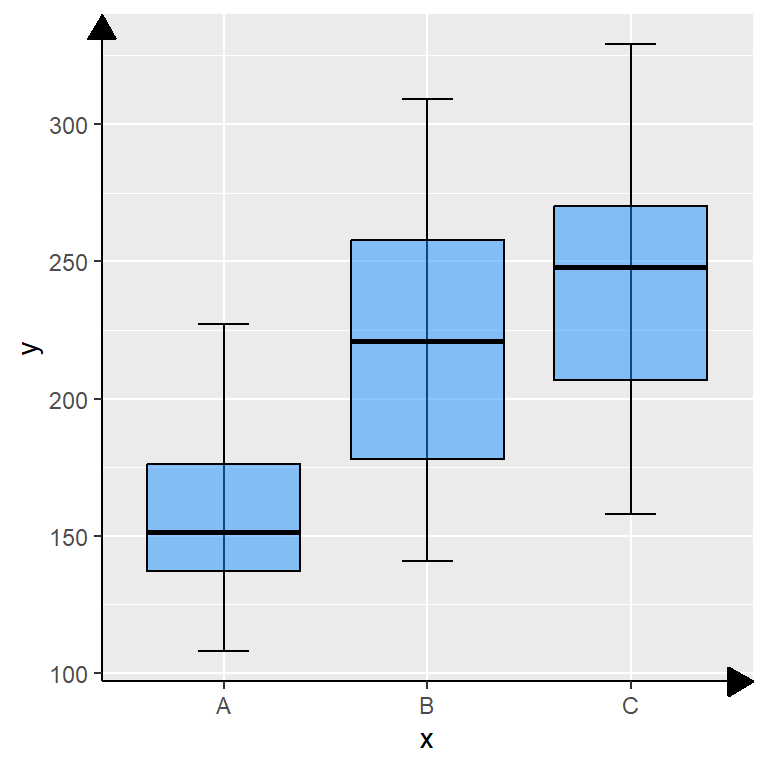Adding arrows to the axis lines

Arrows can be added to the axis lines passing the `arrow` function to the `arrow` argument of the `element_line` function. You can customize the angle, the length, the position (`"last"`, the default, `"first"` or `"both"`) and the type of arrow (`"open"`, the default or `"closed"`).

``````p +
theme(axis.line = element_line(arrow = arrow(angle = 30,
length = unit(0.15, "inches"),
ends = "last",
type = "closed")))``````

## Dual axis

It is possible to add a dual axis in ggplot2 making use of the `sec.axis` argument from `scale_(x|y)_continuous` and the `sec_axis` function. This function needs a formula or function of transformation and the name of the new axis.

``````ggplot(cars, aes(x = speed, y = dist)) +
geom_col() +
geom_smooth(data = cars, aes(x = speed, y = dist * 2)) +
scale_y_continuous(sec.axis = sec_axis(trans = ~.* 2, name = "Z-axis title"))``````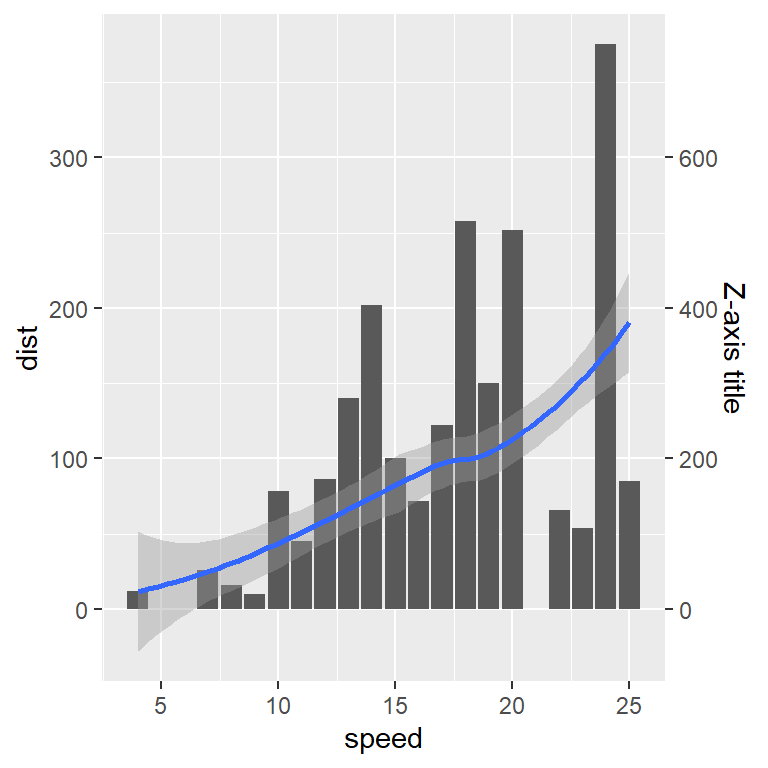Secondary axis breaks

The function also provides the `breaks` argument to customize the default breaks or tick marks of the secondary axis. In the following example we are placing only three tick marks.

``````ggplot(cars, aes(x = speed, y = dist)) +
geom_col() +
geom_smooth(data = cars, aes(x = speed, y = dist * 2)) +
scale_y_continuous(sec.axis = sec_axis(~.* 2, name = "Z-axis title",
breaks = c(100, 200, 300)))``````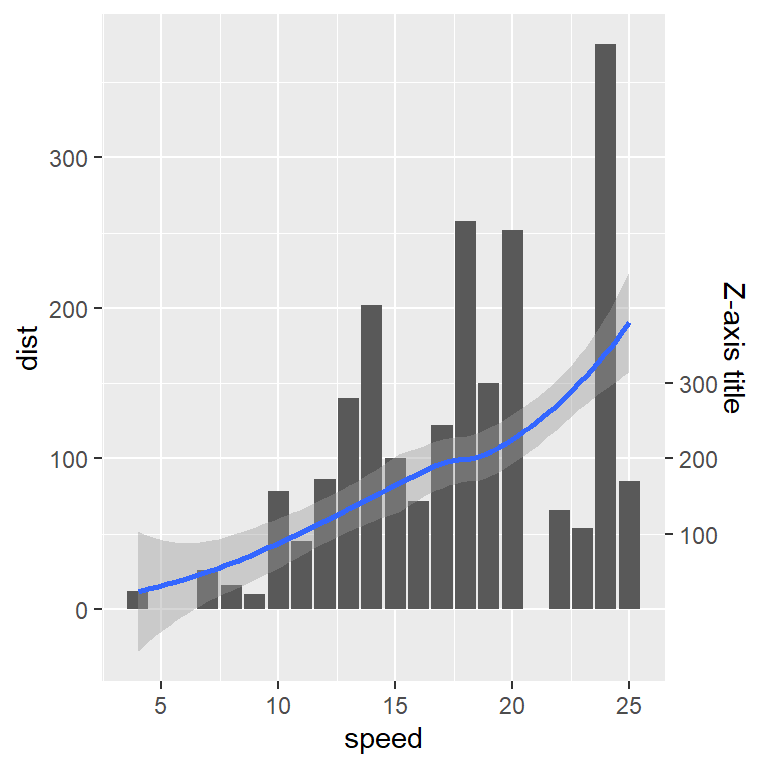Secondary axis labels

The axis labels of the dual axis plot can be customized through the `labels` argument. Remember that the length of `breaks` must equal the length of `labels`.

``````ggplot(cars, aes(x = speed, y = dist)) +
geom_col() +
geom_smooth(data = cars, aes(x = speed, y = dist * 2)) +
scale_y_continuous(sec.axis = sec_axis(~.* 2, name = "Z-axis title",
breaks = c(100, 200, 300),
labels = c("A", "B", "C")))``````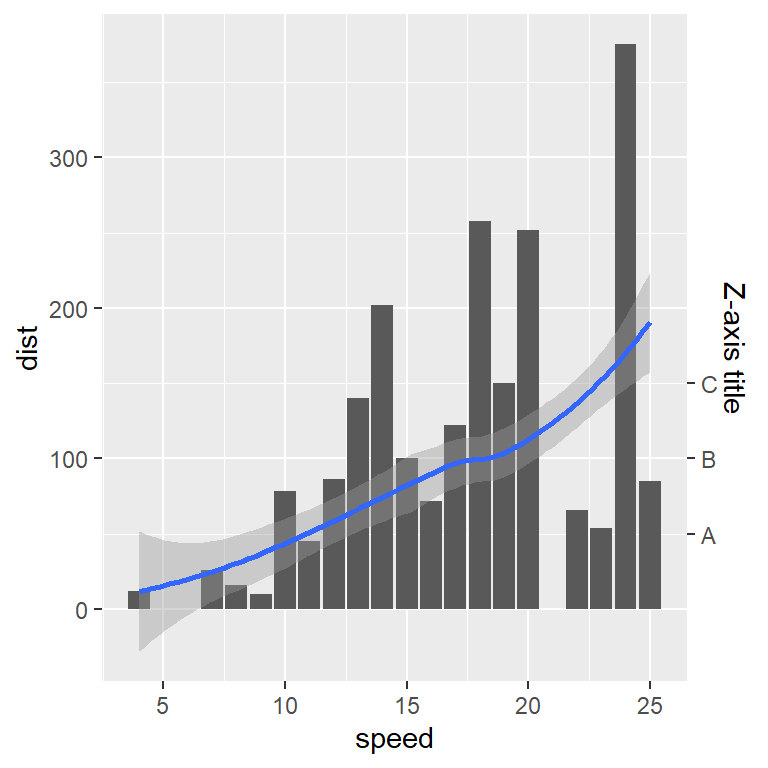Further customization of the dual axis plot

The right axis can be customized making use of the corresponding components. With `axis.title.y.right` you can style the axis title text, such as its color or size, with `axis.text.y.right` the color and size of the secondary axis labels and with `axis.line.y.right` you can customize the axis line.

``````ggplot(cars, aes(x = speed, y = dist)) +
geom_col() +
geom_smooth(data = cars, aes(x = speed, y = dist * 2)) +
scale_y_continuous(sec.axis = sec_axis(~.* 2, name = "Z-axis title")) +
theme(axis.title.y.right = element_text(color = "red",
size = 15,
face = "bold"),
axis.text.y.right = element_text(color = "blue", size = 10),
axis.line.y.right = element_line(color = "orange"))``````